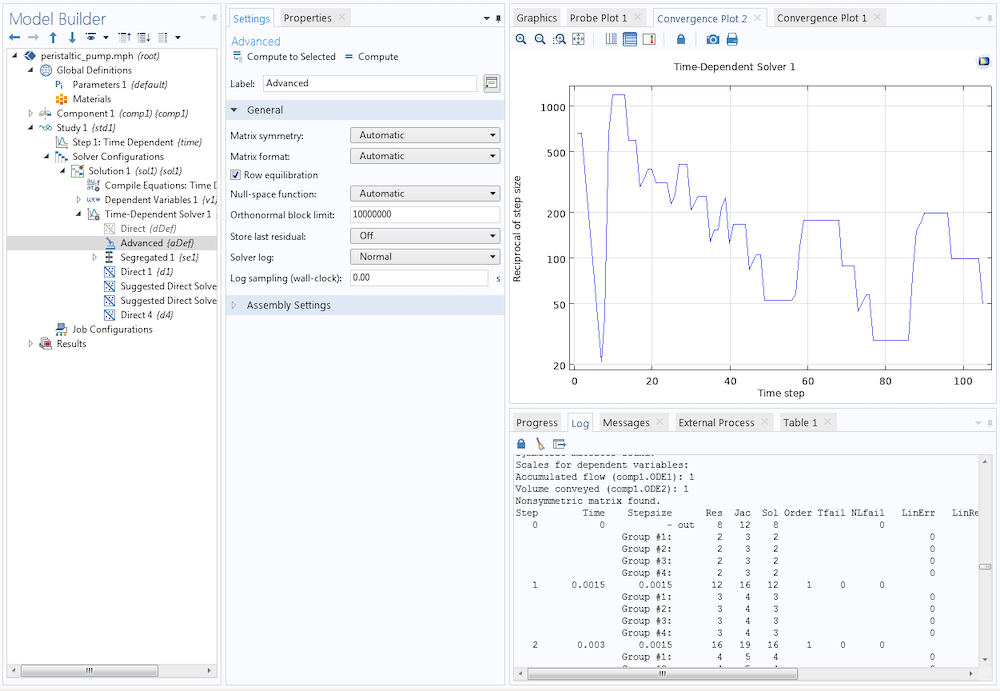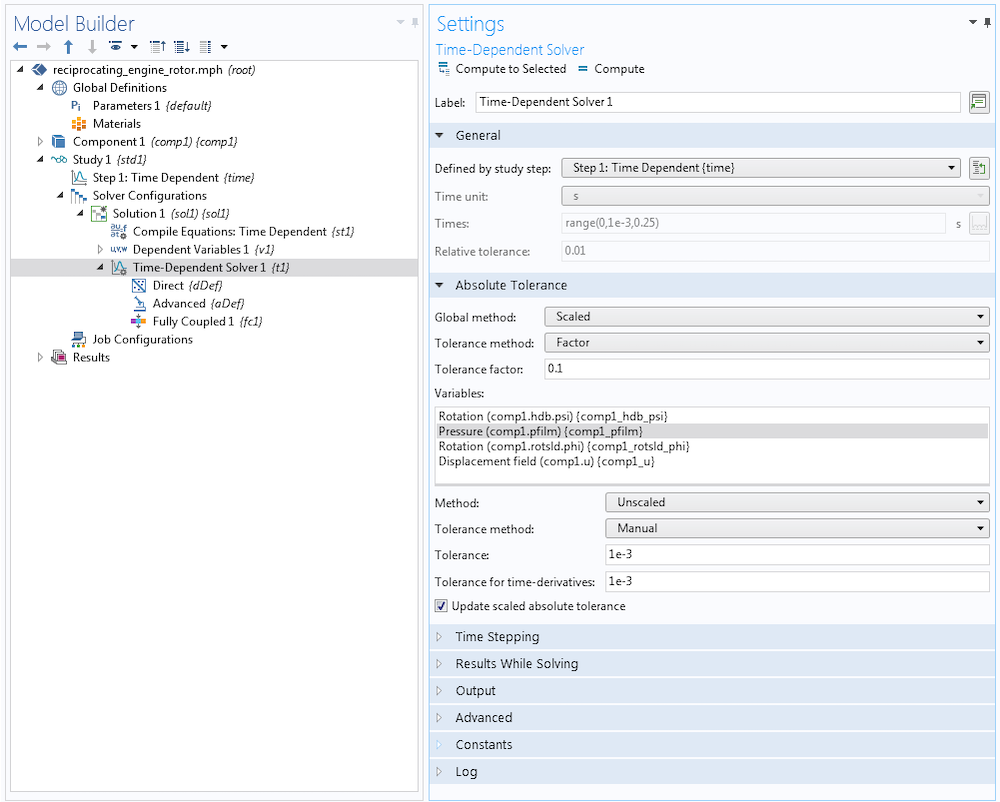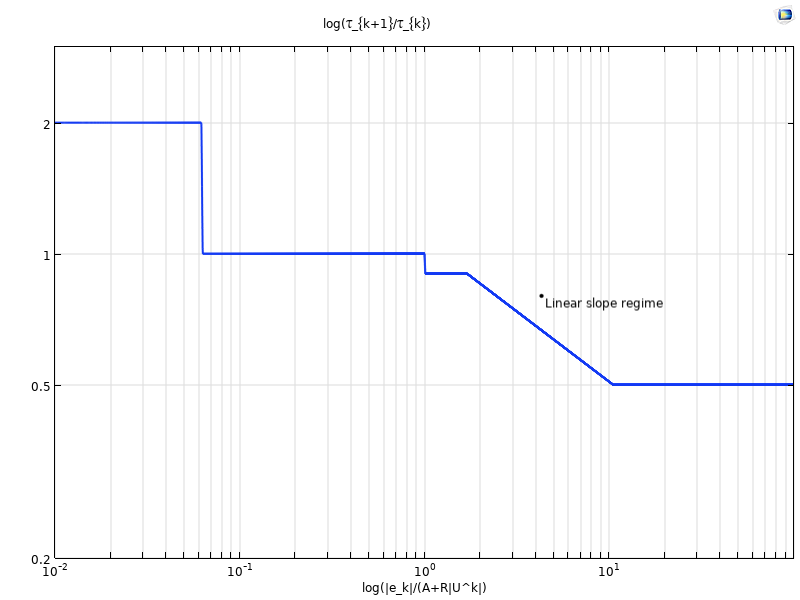# 瞬态问题中的自动时步和阶数选择

2019年 6月 20日

### 瞬态求解器日志

Stepsize Order Tfail NLfail -out Step Time Res Jac Sol LinErr LinRes 0 0 2 3 2 8e-14 3.5e-15 1 0.1 11 5 11 2.6e-16 2.4e-16 ... ... ... ... ... ... ...### 离散时间步进方案

COMSOL 软件中的瞬态求解器 提供了三种不同的时间步进方法：隐式向后差分公式BDF)，广义α方法，以及显式龙格-库塔方法。

BDF 求解器是一种隐式求解器，它使用具有可变离散化阶数和自动步长选择的向后差分公式。当解的质量允许时使用高阶方案，当需要额外的鲁棒性时使用低阶方案。BDF 方法的稳定性是众所周知的，默认情况下，它们用于涉及扩散、对流和反应的问题。### 设置时步选择

• 自由: 基于局部误差估计选择自适应时步。基于当前误差估计与容差的比较，减少或增加时步。如果所采取的时步仍然不满足误差估计，那么该步骤用减小的时步重新计算(Tfail 记录在求解器日志中)。由于失败的步骤是无效的，因此这种步长减小的代价很高。如果非线性求解器循环没有在最大迭代次数内收敛( NLfail 记录在求解器日志中)，指定的输出时间与求解器采用的时步无关。指定输出时间的解通过求解器所采取的时步之间的插值来计算。
• 精确: 与自由 设置相同，但求解程序会调整其时步，也针对指定的输出时间进行求解。
• 中级:与自由设置相同，但求解程序会调整其时步，在由输出时间定义的每个子区间中至少求解一次。
• 手动:采用用户指定的时步，而不是自动的。时步可以是常数、全局变量表达式，或者由时间值的单调列表之间的间隔确定。因此，该时步完全由用户控制，并且该时步的误差测试被禁用。但请注意，代数求解器的误差估计仍然有效，因此容差仍然是需要考虑的重要因素。如果在最大迭代次数后没有达到代数误差估计，则时步减小。

### 局部和全局截断误差以及离散化误差

L(\dot{U},U,t) = 0, \ \ U(0) = U^0

U^k \approx \sum_{i=1}^q \alpha_{k,i} U^{k-i} + \tau_k \beta_k \dot{U}(t_k)

L\left(\frac{U^k-\sum_{i=1}^q \alpha_{k,i} U^{k-i}}{\tau_k \beta_k},U^k,t_k\right) = 0.

L(\dot{U}(t_k;U^{k-1})+O(e_k/\tau_k) + O(\tau_{k}^q), U(t_k;U^{k-1})+e_k,t_k)=0,

L(\dot{U}(t_k;U^{k-1}), U(t_k;U^{k-1}),t_k)+\frac{\partial L}{\partial \dot{U}}(O(e_k/\tau_k) + O(\tau_{k}^q)) + \frac{\partial L}{\partial U}e_k + {\rm h. o. t.}= 0,

|e_k| = O( \tau_k^{q+1} )

U^{k(0)} = \sum_{i=1}^q \alpha_{k,i}^{\rm pred} U^{k-i} + \tau_k \beta_k^{\rm pred} \dot{U}(t_{k-1}).

U^k – U^{k(0)} = \bar{C}_k \tau ^{q+1} U^{(q+1)}(t_k) + O(\tau^{q+2})

|e_{k}| \approx |\bar{e}_k| := \frac{C_k}{\bar{C}_k} |U^k-U^{k(0)}|.

### BDF 时间步进方案的时间步进控制

\|\bar{e}_{k}\|^2_{\rm WRMS} := \frac{1}{M} \sum_j \frac{1}{N_j} \sum_i \frac{ |\bar{e}_{k,i}(q)|^2}{(A_i + R |U^{k}_i|)^2} < 1

1. 时步的大小，\tau_k
2. 离散化的阶数 q

### 时间步长选择和阶数选择\tau^{q+1} U^{(q+1)} \approx T(q) := (q+1) \bar{e}_k.

\tau’，至少 \bar{e}_{k,\tau’} = A+R|U| 是成立的，这就产生了新的时步 \tau’。通过

\frac{A+R|U|}{\bar{e}_{k,\tau}}= \frac{\bar{e}_{k,\tau’}}{\bar{e}_{k,\tau}} = \left(\frac{\tau’}{\tau} \right)^{q+1} < 1

\tau’ =\left(\frac{A+R|U|}{\bar{e}_{k,\tau}}\right) ^{1/(q+1)} \tauT(q) 随着 q 增加，阶数 q 减少。更多细节和启发可以在下列文档中找到：IDA solver in the Suite of Nonlinear and Differential/Algebraic Equation Solvers (SUNDIALS)

### 参考文献

1. G. Söderlind, L. Wang, “Adaptive time-stepping and computational stability“, J. Comp. and Appl. Math., vol. 185, pp. 225–243, 2006.